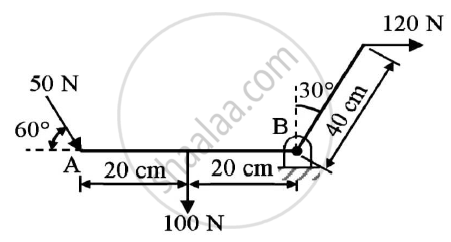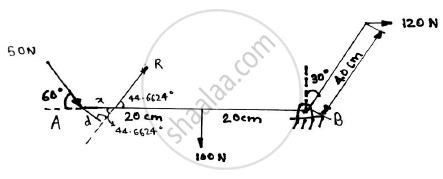# Find the Resultant of the Force Acting on the Bell Crank Lever Shown. Also Locate Its Position with Respect to Hinge B. - Engineering Mechanics

Find the resultant of the force acting on the bell crank lever shown. Also locate its position with respect to hinge B.Given : Forces on the bell crank lever
To find : Resultant and it’s position w.r.t hinge B

#### Solution

Let the resultant of the system of forces be R and it is inclined at an angle θ to the horizontal The hinge is in equilibrium Taking direction of forces towards right as positive and towards upwards as positive
Applying the conditions of equilibrium
ΣFx = 0
Rx = 50cos60 + 120
= 145 N
Ry = -50sin60 -100
= -143.3013
R= sqrt(R2/x+R2/y)
=sqrt(145^2+(-143.3013))^2
= 203.8633 N
θ = tan-(Ry)/(Rx)

=tan^-1((143.3013)/145)
=44.6624oLet the resultant force R be acting at a point x from the point A and it is at a perpendicular distance of d from point A
Taking moment of forces about point A and anticlockwise moment as positive
Applying Varigon’s theorem,
203.8633 x d = -(100 x 20) – (120 x 40cos30)
d = -30.2012 cm = 30.2012 cm ………..(as distance is always positive)
sin 44.6624 =x/30.2012

x = 21.2293 cm
Distance from point B = 40 – 21.2293
=18.7707 cm
Resultant force = 203.8633 N ( at an angle of 44.6624o in first quadrant)
Distance of resultant force from hinge B = 18.7707 cm

Concept: Resultant of parallel forces
Is there an error in this question or solution?